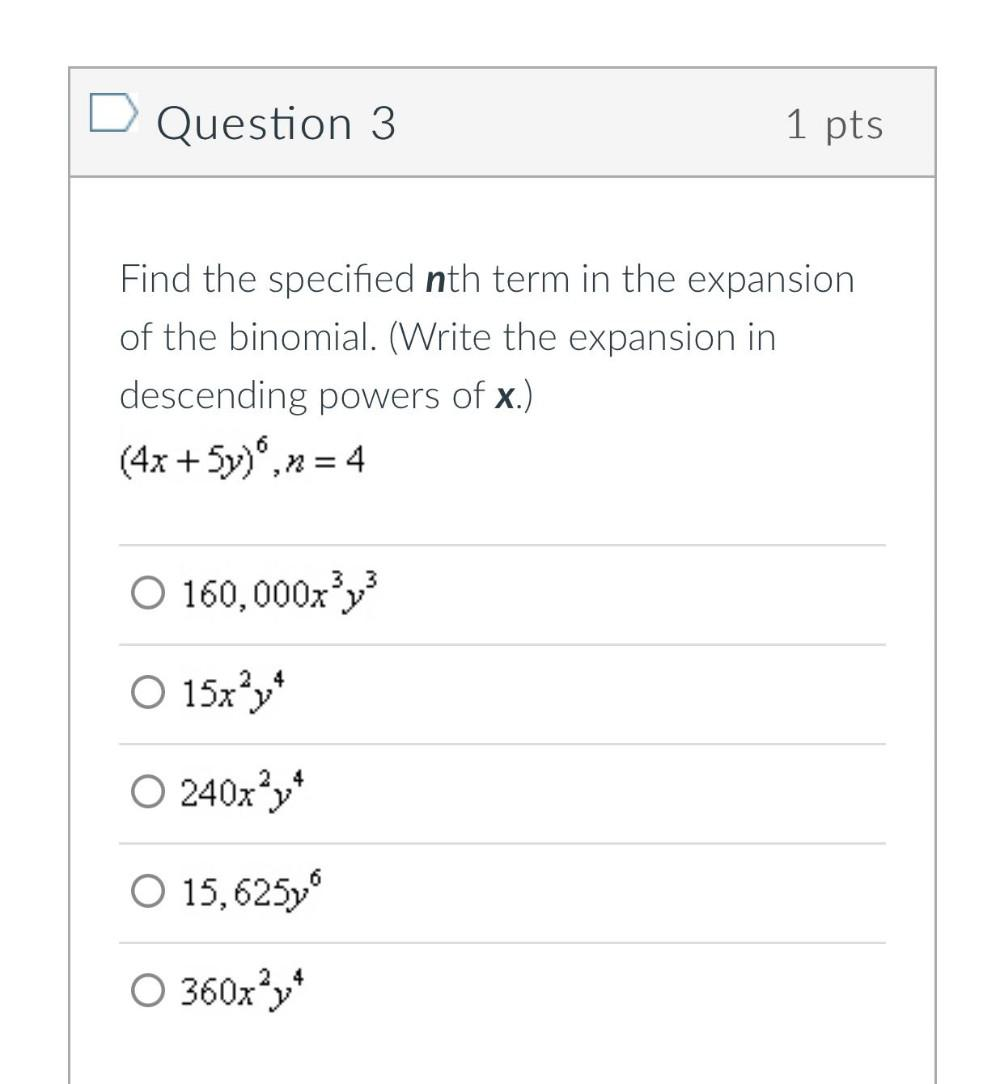Question:

# Find the specified nth term in the expansion of the binomial. (Write the expansion in descending powers of x.) (4x + 5y)^6 ,n=4 a)160,000x^3y^3 b)15x^2y^4 c)240x^2y^4 d)15,625y^6 e)360x^2y^4Find the specified nth term in the expansion of the binomial. (Write the expansion in descending powers of x.) (4x + 5y)^6 ,n=4 a)160,000x^3y^3 b)15x^2y^4 c)240x^2y^4 d)15,625y^6 e)360x^2y^4